taoCMS是基于php+sqlite/mysql的国内最小（100Kb左右）的功能完善的CMS管理系统

## Python机器学习方法智能识别亚马逊验证码

2021-07-16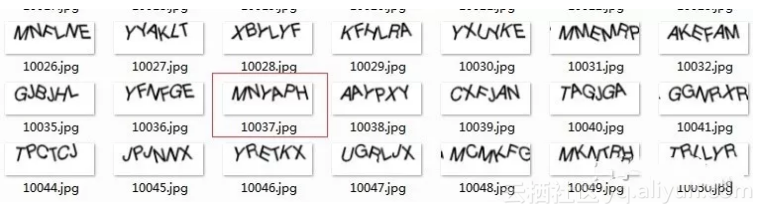1.将原图片作二值化等特殊处理转换得到低像素图片

2.分割出每个字母的图片，并加入到训练库中

3.每个字母图片在训练库中训练

4.将每个字母图片训练后的结果依次组合起来，就是最终验证码

PIL ：图片处理库

scipy ： 科学计算库

●  原图片处理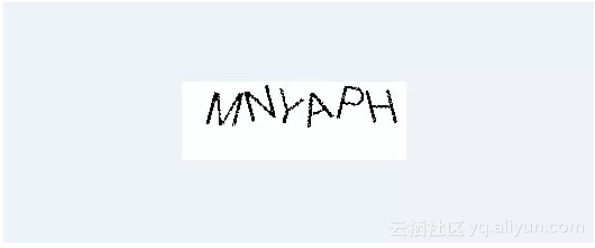``````
``````
`im = Image.open(image_file)`

`im = im.convert('P')`

`im_size = im.size`

`new_im = Image.new('P', im_size, 255)`

`im_width = im_size`
`im_height = im_size`

`for y in range(im_height):`
`for x in range(im_width):`
`pixel = im.getpixel((x, y))`
`if pixel ==0:`
`new_im.putpixel((x, y), pixel)`
●  切割图片得到单个字母图片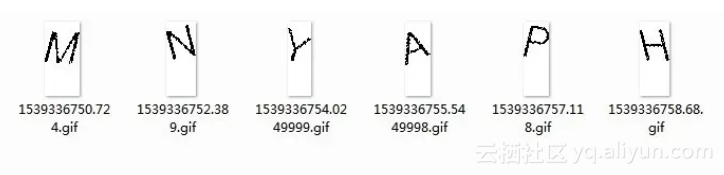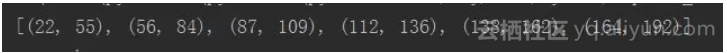``````
``````
`def get_x_coord(image) -> '返回切割的x坐标':`
`image_width = image.size`
`image_height = image.size`

`crop_list = []`
`start_pos = 0`
`is_start_one_char = False`

`for x in range(image_width):`
`is_black_pos = False`
`for y in range(image_height):`
`pixel = image.getpixel((x,y))`
`if pixel == 0:`
`if is_start_one_char == False:`
`start_pos = x`
`is_black_pos = True`
`is_start_one_char = True`
`break`
`if is_start_one_char== True and is_black_pos == False:`
`end_pos = x`
`is_start_one_char = False`
`crop_list.append((start_pos, end_pos))`

`return crop_list`
●  单个字母图片加入到训练库中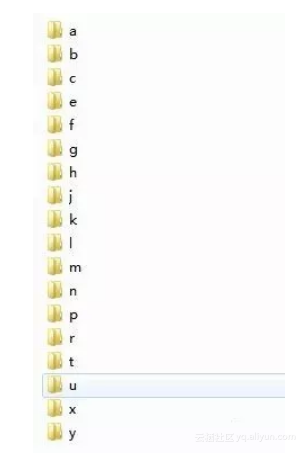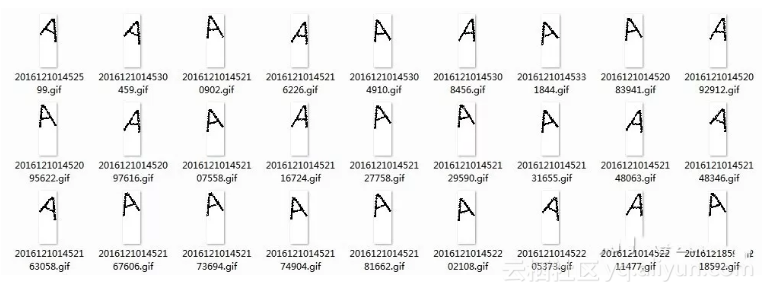●  训练识别单个字母图片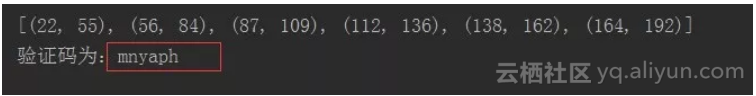``````
``````
`match_captcha = []`
`for crop in crop_list:`
`crop_im = new_im.crop((crop, 0, crop, im_height)) #（左上x， 左上y， 右下x， 右下y）`
`filename = 'e:/crop/' + str(time.time()) + '.gif'`
`# crop_im.save(filename)`

`all_result = [] #单个切片的所有字母的相似性`

`remove_letter = ['d', 'i', 'o', 'q', 's', 'v', 'w', 'z']`
`for letter in list(set(string.ascii_lowercase)- set(remove_letter)):`

`refer_image_dir = r'E:training_library%s' % letter`

`for refer_image in os.listdir(refer_image_dir):`
`refer_im = Image.open(os.path.join(refer_image_dir, refer_image))`

`crop_list = list(crop_im.getdata())`
`refer_list = list(refer_im.getdata())`
`min_count = min(len(crop_list), len(refer_list))`

`result = 1 - spatial.distance.cosine(crop_list[:min_count-1], refer_list[:min_count-1])`
`all_result.append({'letter' : letter, 'result' : result})`

`match_letter = max(all_result, key=lambda x: x['result']).get('letter')`
`match_captcha.append(match_letter)`

`print('验证码为：{0}'.format(''.join(match_captcha)))`

### 公告

taoCMS发布taoCMS 3.0.2(最后更新21年03月15日)，请大家速速升级，欢迎大家试用和提出您宝贵的意见建议。### 捐助与联系☟请使用新浪微博联系我☟

☟在github上follow我☟